##### Hot Keywords

TopIntell Robot 2021;1(2):131-50. 10.20517/ir.2021.09 © The Author(s) 2021.
Open Access Research Article

# Autonomous navigation in unknown environment using sliding mode SLAM and genetic algorithm

Departamento de Control Automatico, National Polytechnic Institute, Mexico City 07360, Mexico.

Correspondence Address: Prof. Wen Yu, Departamento de Control Automatico, National Polytechnic Institute, Mexico City 07360, Mexico. E-mail: yuw@ctrl.cinvestav.mx

This article belongs to the Special Issue Evolutionary Machine Learning for Engineering Applications
Views:915 | Downloads:140 | Cited:0 | Comments:0 | :3
Academic Editors: Simon X. Yang, Hao Zhang | Copy Editor: Yue-Yue Zhang | Production Editor: Yue-Yue Zhang

## Abstract

In this paper, sliding mode control is combined with the classical simultaneous localization and mapping (SLAM) method. This combination can overcome the problem of bounded uncertainties in SLAM. With the help of genetic algorithm, our novel path planning method shows many advantages compared with other popular methods.

## 1. Introduction

### 1.1. Autonomous navigation in unknown environment

Autonomous navigation (AN) has three jobs.

1. Perception: Mapping from signal to information is the perception of AN. Its algorithms can use human thought, intelligent methods, optimization, probability methods, and genetic algorithms.

2. Motion planning: It has three classes, namely graph methods such as a roadmap, random sampling, and grid.

3. Localization and mapping: In unknown environments, sensors, actuators, and maps may have big uncertainties.

Path planning (PP) can be performed under the following conditions:

(1) The environment is known. PP is an optimization problem.

(2) The environment is partially known. PP can find new objects during navigation[14,15].

(3) The environment is totally unknown. PP depends on the navigation and has a recursive solution.

Simultaneous localization and mapping (SLAM) can be used in unknown environments or in partially unknown environments. SLAM uses the current position to construct a map, and it can be classified into feature-based, pose-based, appearance-based, and variants.

The most popular SLAM uses Kalman filter for Gaussian noise. Nonlinear SLAM uses extended Kalman filter (EKF), where the noise assumptions are not satisfied. EKF-SLAM applies linearization.

### 1.2. Related work

Few AN uses SLAM. Visual SLAM uses several cameras. AN can use both SLAM and GPS signals. Robots can avoid moving obstacles using neural networks. Swarm optimization helps robots follow an object. Neural networks help robots construct the navigation path. The optimal path is considered in the sense of trajectory length, execution time, or energy consumption.

Genetic algorithms (GA) have been developed recently[34,35]. They are easy to use for optimization in non-deterministic cases, uncertainty models, and robust cases. GA can be in form of ant-based GA[39,40], cell decomposition GA, potential field GA, ant colony, and particle swarm optimization. Finite Markov chain is a theory tool for GA[45,46].

### 1.3. Our work

In this paper, we try to design AN in an unknown environment in real time. The contributions are as follows:

(1) Sliding mode SLAM: The robustness of this SLAM is better than other SLAM models in bounded noise.

(2) GA SLAM: We use roadmap PP and GA to generate the local optimal map.

(3) Comparisons and simulations with other SLAM models were made by using a mobile robot.

## 2. Sliding mode slam

SLAM gives the robot position and environment map at the same time. At time k, the state is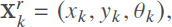where (xk, yk) is the position and θk is the orientation of the robot.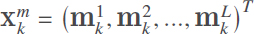are landmarks, with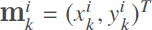the ith landmark. We assume the true location is time-invariant.

Xk has two parts: the robot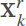and the landmarks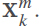The state equation is

where f () is the robot dynamics, Wk is the noise, and uk is the robot control. Since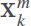is not influenced by motion noise, the noise is [Wk, 0]T.

Zk is defined as the position between the robot and the landmark, whose model is

where h() is the geometry and vik is the noise. Here, wk and vik are not Gaussian noises. We assume wk and vik are bounded.

To estimate xk in Equations (1) and (2), EKF is needed. We linearize the state model in Equation (1) and the observation model in Equation (2) as

where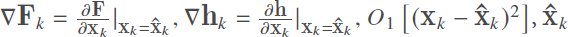is the estimation of xk.

Prediction. The estimation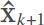is based on past states, control, and landmarks:

where R1 is the covariance of Wk, R1 = E {[WkE (Wk)] [Wk – E (Wk)]T}.

Correction. The new state is based on predicted states, landmarks, and current observations:

The motivations of using sliding mode modification to the EKF bases SLAM based are the following:

(1) The noises wk and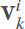in Equations (1) and (2) are not Gaussian.

(2) There are linearization error terms,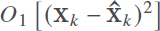and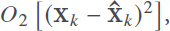in Equation (3), and the traditional EKF-based methods do not work well for these errors.

We use the sliding mode method to estimate the robot state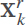and the landmark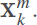Sliding modes have a number of attractive features, and thus have long been in use for solving various control problems. The basic idea behind design of system with sliding mode is the following two steps: (1) a sliding motion in a certain sense is obtained by an appropriate choice of discontinuity surfaces; and (2) a control is chosen so that the sliding modes on the intersection of those discontinuity surface would be stable. A general class of discontinuous control u (x, t) is defined by the following relationships:

where the functions u+ (x, t) and u (x, t) are continuous.

The function S (x) is the discontinuity surface (subspace). The objective of the sliding mode control is to design some switching strategy of the continuous control u+ (x, t) and u (x, t), such that

In this paper, the sliding surface is defined by the SLAM estimation error as

Here, the discontinuity surface is e (k) = [e1… en]. We consider the following positive definite function,

where P is diagonal positive definite matrix, P = PT > 0. The derivative of V is

The motion e (k) satisfies

where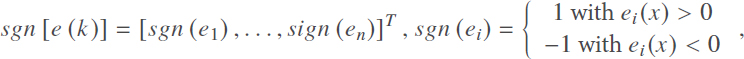sgn (0) = 0, then (10) is

because P = diag {pi}, pi > 0, and ei × sgn (ei) = |ei|

Thus,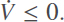By Barbalat’s lemma, the estimation error is e (k) → 0.

The classical SLAM in Equations (4) and (5) is modified by the sliding surface in Equation (11). The sliding mode control can be regarded as a compensator for Equation (4):

where ρ is a positive constant. The correction step is the same as EKF in Equation (5). The sliding mode SLAM is shown in Figure 1. Here, the estimation error, e (k), is applied to the sliding surface to enhance the robustness in the prediction step with respect to the noise and disturbances.

It is the discrete-time version of Equation (6). We give the stability analysis of this discrete-time sliding mode SLAM at the end of this section.

For the mobile robot, the sliding mode SLAM can be specified as follows. We define a critical distance dmin to limit the maximal landmark density. It can reduce false positives in data association and avoid overload with useless landmarks. If the new landmark is far from the other landmarks on the map, then the landmark is added; otherwise, it is ignored. If the distance between the new landmark Xk+1 = [xm+1,ym+1] and the others is bigger than dmin, it should be added into xk, i.e.,

It can be transformed into an absolute framework as

The nonlinear transformation function T also applies to the uncertainties. We approximate the transformation T by the linearization. Pk can be expressed as

where

with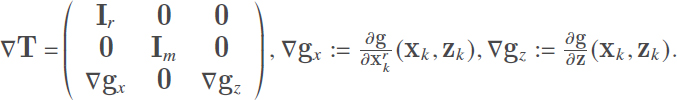For the motion part, we use the Ackerman vehicle model 

where Wk is the process noise, vk is the linear velocity, αk is the steering angle, Tk is the sample time, and ba is the distance between the front and the rear wheels.

At the beginning of map building, the vector k only contains the robot states without landmarks. As exploration increases, the robot detects landmarks and decides if it should add these new landmarks to the state.

where x, y, z, and m are defined in Equations (1) and (2),

We exploit the same property in the sliding SLAM. The landmarks with fewer corrections are removed from the state vector.

where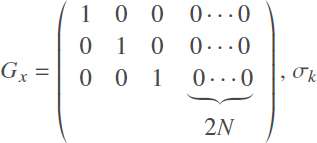is the compensator, and

This sliding SLAM algorithm is given in the following algorithm.

The discrete-time sliding mode SLAM in Equation (19) can be written as

where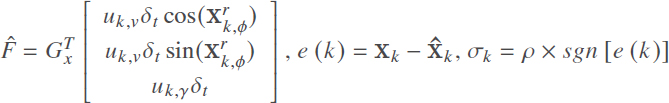The correction step for k+2 is the same as EKF:

where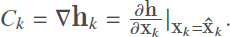The error dynamic of this discrete-time sliding mode observer is

where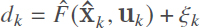is bounded uncertainty,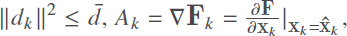and Kk is the gain of EKF in Equation (21).

The next theorem gives the stability of the discrete-time sliding mode SLAM.

Theorem 1If the gain of the sliding mode SLAM is positive, then the estimation error is stable, and the estimation error converges to

where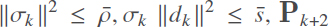is the gain of EKF in Equation (21),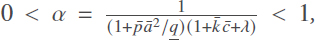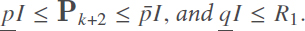Proof 1Consider the Lyapunov function as

wherePk+2is the prior covariance matrix in Equation (21), andPk+2> 0. From Equation (22),

Because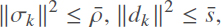the last term on the right side of Equation (25) is

where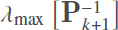is the maximum eigenvalue ofThe second term of Equation (25) is

whereKkis the gain of EKF in Equation (5). In view of the matrix inequality

which is valid for any X,Y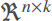and for any positive definite matrix 0 < Λ = ΛT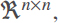the first term of Equation (27) is

We apply the sliding mode compensation in Equation (20) to the second term of Equation (27):

where lij are the elements of the matrix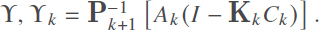When the the orientation θk is not big sin θk 0, cos θk 0,

Thus, the second term of Equation (27) is negative.

The first term on the right side of Equation (25) has the following properties:

(I - KkCk) is invertible, and we have

According to EKF,

Thus,

By the following matrix inversion lemma,

where Γ and Ω are two non-singular, matrices,

Using Equation (32) and defining L = (1 –KkCk),

Now,

Hence,

Combining the last term of Equation (29) with the first term on the right side of Equation (25),

where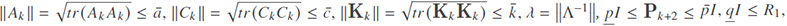and

Combining Equation (26), the first term of Equation (29), and Equation (34),

Thus,

where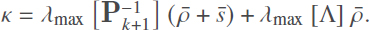If

then Vk+1– Vk ≤ 0, ||e (k)|| decreases. Thus, ||e (k)|| converges to Equation (23).

## 3. Genetic algorithm and slam for path planning

Path planning is one key problem of autonomous robots. Here, the map is built by the sliding mode SLAM:

• The obstacle set is defined by Bobs(t).

• The position is xr(t), Bfree(t) = B\Bobs(t).

• The path planning is f (x (t), xs, xT),xs = xr(t).

The previous map is Bfree(t), which requires the path f(x(t),xs,xT).

We assume the previous map is obstacle-free, the initial point is xs, the target point is xTB,

the obstacle is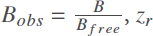is the shape of the robot, and A(zr) is the area of the robot. The objective of the path planning is to find a path f(x,xs,xT)Bfree that allows the robot to navigate.

D is defined as the search space. We use the GA to find an optimal trajectory f (x, xs, xT), such that

Here, we use stochastic search for GA, and each iteration includes: reproduction or selection, crossing or combination, and mutation. The population iswith m being the size of the population that represents the possible solutions:

(1) Every chromosome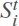has a solution in D.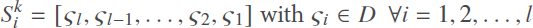(2) Crossing the chromosomes. An intersection in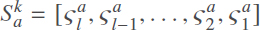and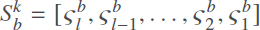belongs to D, such that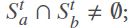then,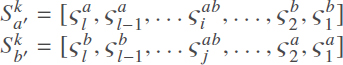where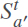and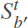are the next generation from two compatible chromosomes by crossing.

(3) Mutation. It replace a number of chromosomes by chromosomes in D.

The mutation operation is calculated by the fitness of each chromosome,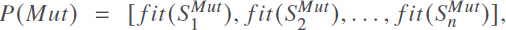where n is the number of mutations and fit uses the

Euclidean distance. The total fitness is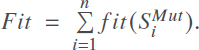Therefore, the probability of selection pi, of a chromosome Si for i = 1, 2,…, n is

An optimal solution pM in D is mutated by

In the mutation operation, an optimal solution with pM = 1 is a global solution if n ∞. To prove the convergence, we use a Markov chain, as shown in Figure 2. Each chromosome can move from Qij to the state Qi(j+1). The moving probability is ρji,ik > 0, i = 1, 2, …,n, k,j = 1, 2, …,m.

The operators, selection, crossing, and mutation create P(k) with pk. It preserves the best chromosomes of P(k – 1). P(k + 1) in the population P(k) can be regarded as the Markov transition:

Theorem 2If GA for the roadmap is an elitist process, then the probability of p*in D is exponential.

Proof 2The iteration Q1 is changed with the chromosomes when genetic process is elitist,

where n is the size of the population. If for all α, βD, there is 0 < τ ≤ msuch that Hτ (α, β) ≥ ∊ > 0, then

This implies that, given certain state Qt, the probability of transition in time t between t and t + m is at least ∊,

Without loss of generality, the transition in the iteration k + 1 is

Using Equation (42), we have

where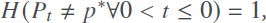then

Since 0 <≤ 1, the algorithm converges exponentially to p* in: population size n and iteration number k.

The algorithm of the SLAM-based roadmap GA for the path planning is as follows.

Our AN uses both sliding mode SLAM (Algorithm 1) and the roadmap GA method (Algorithm 2). The autonomous navigation algorithm is:

The PP needs the map, robot position, and target. This information is given by the sliding mode SLAM algorithm. When the algorithm falls into a local solution, we use the ComputeOutsideLocal function to provide another Sn which is outside the local zone.

## 5. Comparisons

In this section, we use several examples to compare our method with the three other recent methods: the polar histogram method for path planning, the grid method for path planning, and SLAM with extended Kalman filter .

### 5.1. Simulations

The following simulations were implemented in partially unknown and completely unknown environments. The size of the environments was 100 m × 100 m, in which a solution was sought to find a trajectory from the initial point xs to the target point xT. The sliding mode gains were selected as ρ = diag ([0.1, …, 0.1]).

In the partially unknown environments, Bobs(0) ≠ θ. The path planning solution p* was partial because the environment Bobs(t) was variant in time. Figure 3 shows a partial solution p* from an initial point xs to the objective point xT for the partially unknown environment. Figure 4 shows the overall result of the robot navigation from point xs to point xT with the robust SLAM algorithm combined with the GA.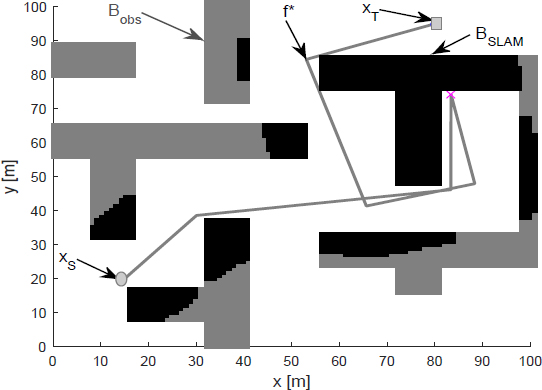Figure 4. Autonomous navigation using sliding mode simultaneous localization and mapping and genetic algorithm method.

Here, the SLAM algorithm was used to construct the environment and find the position of the robot. At the beginning of navigation in the partially unknown environment, there was a planned trajectory of navigation through the GA algorithm; however, if an obstacle was found in the planned trajectory, the GA algorithm needed to be used to search for a new trajectory within the built environment by the SLAM, BSLAM. The planned trajectory belonged to the set of obstacles that prevent reaching the goal, p* (Bobs(t); therefore, it was necessary to look for a new trajectory using RGA that allowed reaching the goal.

For the completely unknown environments, Bobs(0) = θ. In these environments, the SLAM algorithm was required to know the environment BSLAM and the position of the robot; in this way, when an obstacle was found that contained the planned trajectory, a new trajectory with the GA algorithm was searched on the map

BSLAM until the target point was reached the results obtained are shown in Figure 5. When we used the polar histogram method for path planning , only the local solutions could be found [Figure 6].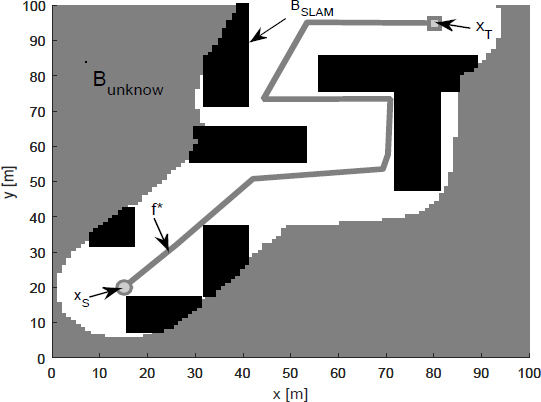Figure 5. Sliding mode simultaneous localization and mapping and genetic algorithm in complete unknown environment.

Now, we compare the path lengths with the polar histogram method. The following density of the obstacles give the navigation complexity. The environment is free of obstacles when dobs = 0. The whole environment is occupied by the obstacles when dobs = 1. The index for the trajectory error is

We use the averages of the path length. The obstacles density is defined as

The path length is defined as

The averages of the path lengths of our RA and the polar histogram are shown in Figure 7. When the density of obstacles was bigger, the path length of the polar histogram grew more quickly than that of ours. When the obstacle density dobs was 0.3, E[lsub, Navigation1] = 1.053, E[lsub, Navigation2] = 1.152.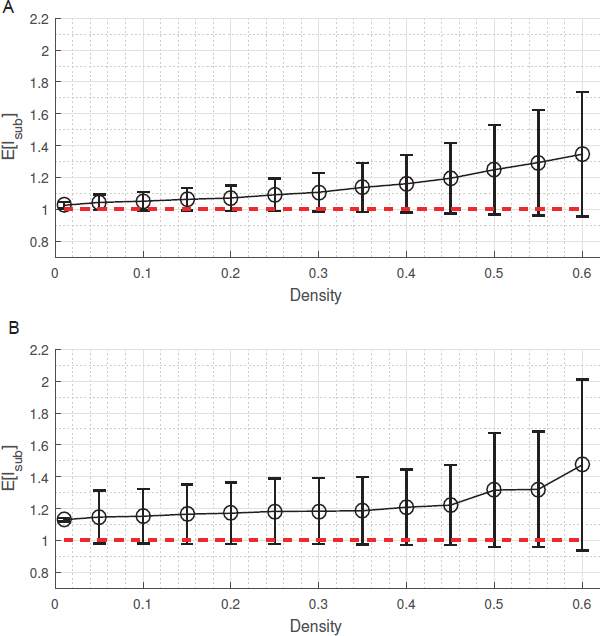Figure 7. The average path length: (A) sliding mode simultaneous localization and mapping and genetic algorithm; and (B) polar histogram.

Next, we compare our method with the grid method. The comparison results are shown in Figure 8. For the task of navigating the robot or system in partially unknown or completely unknown environments, the SLAM algorithm was used to construct the environment and know the position of the robot. At the beginning of navigation in the partially unknown environment, there was a planned trajectory of navigation through the GA algorithm; however, if an obstacle were found in the planned trajectory, the GA algorithm needed to be used to search for a new trajectory within the built environment by the SLAM, BSLAM.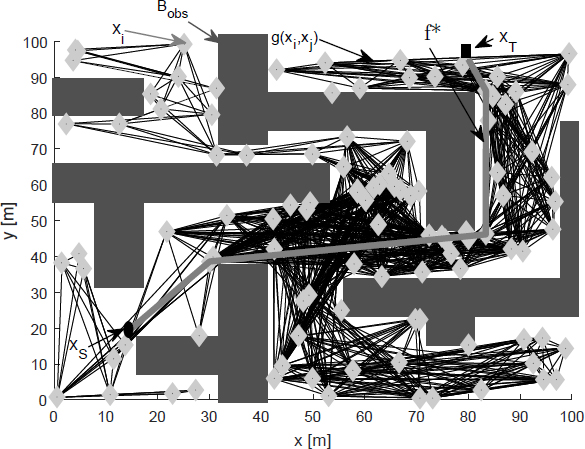Figure 8. Sliding mode simultaneous localization and mapping (gray) and grid method (black)

The size of the environments was 100m× 100 m, in which a solution was sought to find a trajectory from the initial point xs to the target point xT.Figure 9 shows a path planning based on the proposed methods to find a solution f*. Here, 60 local targets were generated xi with the search space D generated by the trajectories of the local targets that do not intersect with the set of obstacles; thus, it became an optimization problem to find an optimal path.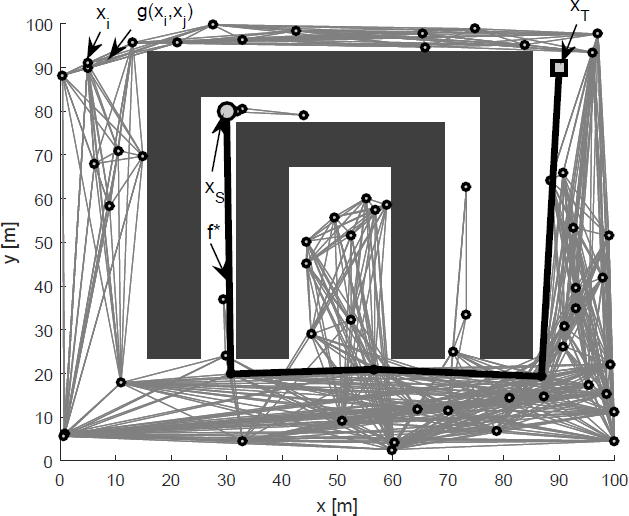Figure 9. Sliding mode simultaneous localization and mapping based path planning (bold) and grid method (gray).

In an environment with previously generated obstacles of 100 m × 100 m, 120 possible targets were randomly generated xi; therefore, the search space D would be all trajectories g(xi,xj) that do not intersect with the set of obstacles, where the roadmap genetic algorithm solved the problem of optimization to find a solution to the problem of path planning. For the problem presented above, we found that the proposed algorithm converged in 40 iterations. For these results, 100 tests were performed for each number of iterations and, as shown in Figure 10, the roadmap genetic algorithm converged with greater probability within 40 iterations.

### 5.2. Application

The Koala mobile robot by K-team Corporation 2013 was used to validate our sliding mode SLAM. This mobile robot has encoders and one laser range finder. The position precision is less than 0.1 m.

The objective of this autonomous navigation is to force the robot to return to the starting point. The sliding mode SLAM was compared with SLAM with extended Kalman filter (EKF-SLAM) 

The initial covariance matrices are zero. The parameters of the algorithm are

Since the robot moves in the environment with bounded noise (see Figure 11), the noises are not Gaussian. Two different conditions are considered: (1) Koala robot pre-processes off-line the sensors data to reduce 90% noises; and (2) the computer uses sliding mode SLAM on-line.

For the first case, Figure 12 shows that sliding mode SLAM and EKF-SLAM are similar. Both sliding mode SLAM and EKF-SLAM work well for the case with less noise. The robot can return to the starting point, and the map is constructed correctly.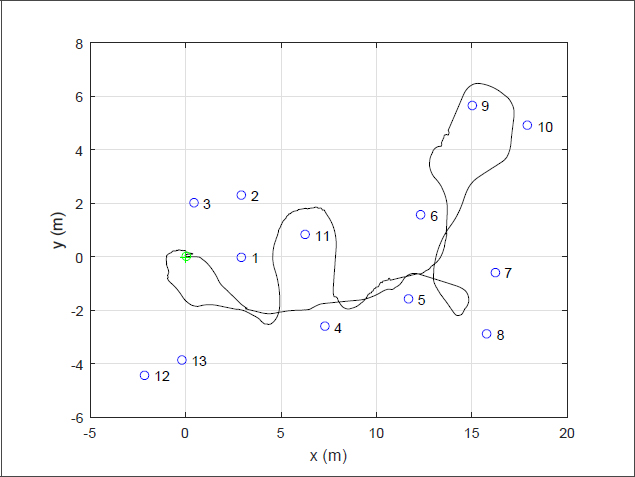Figure 12. Results of extended Kalman filter simultaneous localization and mapping and sliding mode simultaneous localization and mapping with small noises.

For the second case, Figure 13 gives the results of EKF-SLAM. As can be seen, the robot cannot return to the starting point and the map is not exactly the same as the real one with EKF-SLAM because EKF-SLAM is sensitive to non-Gaussian noises.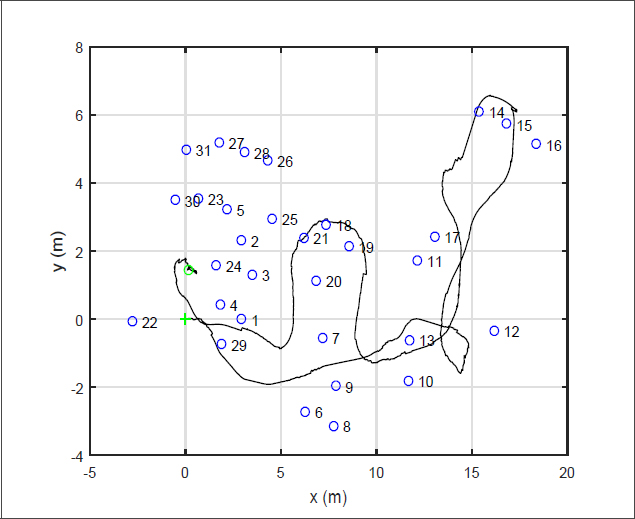Figure 13. Results of extended Kalman filter simultaneous localization and mapping with noises.

Figure 14 shows the results with SM-SLAM. Under the same bounded noises, SM-SLAM works very well, because of the sliding mode technique.

To compare the errors, we define the average of the Euclidean errors as

where NT is the data number;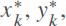and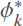are real values for robot position and orientation; and xk, yk, and ϕk are estimations of them. Figure 15 shows the errors of EKF-SLAM and sliding mode SLAM. Obviously, the errors of EKF-SLAM increase quickly. Robots have better estimation in long distances with sliding mode SLAM.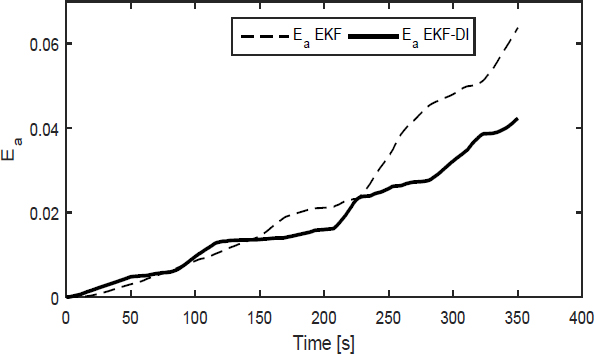Figure 15. Direction estimation errors of sliding mode simultaneous localization and mapping (SLAM) and EKF-SLAM. EKF: Extended Kalman filter.

## 6. Conclusion

Navigation in unknown environments is a big challenge. In this paper, we propose sliding mode SLAM with genetic algorithm for path planning. Both sliding mode and GA can work in unknown environments. Convergence analysis is given. Two examples were applied to compare our model with other models, and the results show that our algorithm is much better in unknown environments.

## Declarations

Authors’ contributions

Revised the text and agreed to the published version of the manuscript: Ortiz S, Yu W

Availability of data and materials

Not applicable.

None.

Conflicts of interest

Both authors declared that there are no conflicts of interest.

Ethical approval and consent to participate

Not applicable.

Consent for publication

Not applicable.

## References

• 1. Luettel T, Himmelsbach M, Wuensche HJ. Autonomous ground vehicles—concepts and a path to the future. Proceedings of the IEEE 2012;100:1831-39.

DOI
• 2. Galceran E, Carreras M. A survey on coverage path planning for robotics. Rob Auton Syst 2013;61:1258-76.

DOI
• 3. Lowe DG. Distinctive image features from scale-invariant keypoints. Int J Comput Vis 2004;60:91-110.

DOI
• 4. Arel I, Rose DC, Karnowski TP. Deep machine learning-a new frontier in artificial intelligence research. IEEE Comput Intell Mag 2010;5:13-8.

DOI
• 5. Scheirer WJ, de Rezende Rocha A, Sapkota A, et al. Toward open set recognition. IEEE Trans Pattern Anal Mach Intell 2012;35:1757-72.

DOI
• 6. Ramos FT, Kumar S, Upcroft B, et al. A natural feature representation for unstructured environments. IEEE Trans Robot 2008;24:1329-40.

DOI
• 7. Lillywhite K, Lee DJ, Tippetts B, et al. A feature construction method for general object recognition. Pattern Recognition 2013;46:3300-14.

DOI
• 8. Chabini I, Lan S. Adaptation of the A* algorithm for the computation of fastest paths in deterministic discrete-time dynamic networks. IEEE trans Intell Transp Syst 2002;3:60-74.

DOI
• 9. LaValle SM, Kuffner JJ Jr. Randomized kinodynamic planning. Int J Rob Res 2001;20:378-400.

DOI
• 10. Valencia R, Andrade-Cetto J. Mapping, planning and exploration with Pose SLAM. Berlin: Springer; 2018. pp. 60-84.

DOI
• 11. Wang X, Shi Y, Ding D, et al. Double global optimum genetic algorithm–particle swarm optimization-based welding robot path planning. Eng Optim 2016;48:299-316.

DOI
• 12. Jones M, Peet MM. A generalization of Bellman’s equation with application to path planning, obstacle avoidance and invariant set estimation. Automatica 2021;127:109510.

DOI
• 13. Rehman NU, Kumar K, Abro GeM. Implementation of an autonomous path plan-ning & obstacle avoidance UGV using SLAM. 2018 International Conference on Engineer-ing and Emerging Technologies (ICEET) 2018. pp. 1-5.

DOI
• 14. de Moura Souza G, Toledo CFM. .

DOI
• 15. Zhang X, Zhao Y, Deng N, et al. Dynamic path planning algorithm for a mobile robot based on visible space and an improved genetic algorithm. Int J Adv Robot Syst 2016;13:91.

DOI
• 16. Clemens J, Reineking T, Kluth T. An evidential approach to SLAM, path planning, and active exploration. Int J Approx Reason 2016;73:1-26.

DOI
• 17. Chen Y, Huang S, Fitch R. Active SLAM for mobile robots with area coverage and obstacle avoidance. IEEE ASME Trans Mechatron 2020;25:1182-92.

DOI
• 18. da Silva Arantes M, Toledo C F M, Williams B C, et al. Collision-free encoding for chance-constrained nonconvex path planning. IEEE Trans Robot 2019;35:433-48.

DOI
• 19. Yu W, Zamora E, Soria A. Ellipsoid SLAM: a novel set membership method for simultaneous localization and mapping. Autonomous Robots 2016;40:125-37.

DOI
• 20. Williams H, Browne WN, Carnegie DA. Learned action slam: sharing slam through learned path planning information between heterogeneous robotic platforms. Appl Soft Comput 2017;50:313-26.

DOI
• 21. Dissanayake MWMG, Newman P, Clark S, et al. A solution to the simultaneous localization and map building (SLAM) problem. IEEE Trans Rob Autom 2001;17:229-41.

DOI
• 22. Thrun S, Liu Y, Koller D, et al. Simultaneous localization and mapping with sparse extended information filters. Int J Robot Res 2004;23:693-716.

DOI
• 23. Folkesson J, Christensen HI. Closing the loop with graphical SLAM. IEEE Trans Robot 2007;23:731-41.

DOI
• 24. Ho KL, Newman P. Loop closure detection in SLAM by combining visual and spatial appearance. Rob Auton Syst 2006;54:740-9.

DOI
• 25. Nieto J, Guivant J, Nebot E. Denseslam: simultaneous localization and dense mapping. Int J Robot Res 2006;25:711-44.

DOI
• 26. Chen SY. Kalman filter for robot vision: a survey. IEEE Trans Ind Electron 2011;59:4409-20.

DOI
• 27. Sibley G, Matthies L, Sukhatme G. Sliding window filter with application to planetary landing. J Field Robot 2010;27:587-608.

DOI
• 28. Kaess M, Johannsson H, Roberts R, et al. iSAM2: Incremental smoothing and mapping using the Bayes tree. Int J Robot Res 2012;31:216-35.

DOI
• 29. Yang S, Scherer SA, Yi X, et al. Multi-camera visual SLAM for autonomous navigation of micro aerial vehicles. Rob Auton Syst 2017;93:116-34.

DOI
• 30. Weiss S, Scaramuzza D, Siegwart R. Monocular-SLAM–based navigation for autonomous micro helicopters in GPS-denied environments. J Field Robot 2011;28:854-874.

DOI
• 31. Zhu A, Yang SX. Neurofuzzy-based approach to mobile robot navigation in unknown environments. IEEE Trans Syst Man Cybern C Appl Rev 2007;37:610-21.

DOI
• 32. Juang CF, Chang YC. Evolutionary-group-based particle-swarm-optimized fuzzy controller with application to mobile-robot navigation in unknown environments. IEEE Trans Fuzzy Syst 2011;19:379-92.

DOI
• 33. Villacorta-Atienza JA, Makarov VA. Neural network architecture for cognitive navigation in dynamic environments. IEEE Trans Neural Netw Learn Syst 2013;24:2075-87.

DOI
• 34. Song B, Wang Z, Sheng L. A new genetic algorithm approach to smooth path planning for mobile robots. Assem Autom 2016;36:138-45.

DOI
• 35. Zhang Y, Gong D, Zhang J. Robot path planning in uncertain environment using multi-objective particle swarm optimization. Neurocomputing 2013;103:172-85.

DOI
• 36. Karami AH, Hasanzadeh M. An adaptive genetic algorithm for robot motion planning in 2D complex environments. Comput Electr Eng 2015;43:317-29.

DOI
• 37. Pereira AGC, de Andrade BB. On the genetic algorithm with adaptive mutation rate and selected statistical applications. Comput Stat 2015;30:131-50.

DOI
• 38. Tsai CC, Huang HC, Chan CK. Parallel elite genetic algorithm and its application to global path planning for autonomous robot navigation. IEEE Trans Ind Electron 2011;58:4813-21.

DOI
• 39. Zhu Q, Hu J, Cai W, et al. A new robot navigation algorithm for dynamic unknown environments based ondynamic path re-computation and an improved scout ant algorithm. Appl Soft Comput 2011;11:4667-76.

DOI
• 40. Arantes MS, Arantes JS, Toledo CFM, et al. A hybrid multi-population genetic algorithm for uav path planning. Proceedings of the Genetic and Evolutionary Computation Conference. 2016 2016. pp. 853-60.

DOI
• 41. Tuncer A, Yildirim M. Dynamic path planning of mobile robots with improved genetic algorithm. Comput Electr Eng 2012;38:1564-72.

DOI
• 42. Raja R, Dutta A, Venkatesh KS. New potential field method for rough terrain path planning using genetic algorithm for a 6-wheel rover. Rob Auton Syst 2015;72:295-306.

DOI
• 43. Arora T, Gigras Y, Arora V. Robotic path planning using genetic algorithm in dynamic environment. Int J Comput Appl 2014;89:9-12.

DOI
• 44. Roberge V, Tarbouchi M, Labonté G. Comparison of parallel genetic algorithm and particle swarm optimization for real-time UAV path planning. IEEE Trans Industr Inform 2012;9:132-41.

DOI
• 45. Bhandari D, Murthy CA, Pal SK. Genetic algorithm with elitist model and its convergence. Intern J Pattern Recognit Artif Intell 1996;10:731-47.

DOI
• 46. Hu ZB, Xiong SW, Su QH, et al. Finite Markov chain analysis of classical differential evolution algorithm. J Comput Appl Math 2014;268:121-34.

DOI
• 47. K-Team Corporation. Available from: http://www.k-team.com/ [Last accessed on 10 Dec 2021].

• 48. Souahi A, Naifar O, Makhlouf AB, et al. Discussion on Barbalat Lemma extensions for conformable fractional integrals. Int J Control 2019;92:234-41.

DOI
• 49. Erdman AG, Sandor GN, Kota S. Mechanism design: analysis and synthesis. Upper Saddle River, NJ: Prentice hall; 2001.

• 50. Wilde N, Kulić D, Smith SL. Learning user preferences in robot motion planning through interaction. 2018 IEEE International Conference on Robotics and Automation (ICRA) 2018. pp. 619-26.

DOI
• 51. Farzan S, DeSouza GN. Path planning in dynamic environments using time-warped grids and a parallel implementation. arXiv.1903.07441 2019.

• 52. Saeedi S, Nardi L, Johns E, et al. Application-oriented design space exploration for SLAM algorithms. 2017 IEEE International Conference on Robotics and Automation (ICRA) 2017. pp. 5716-23.

DOI

Ortiz S, Yu W. Autonomous navigation in unknown environment using sliding mode SLAM and genetic algorithm. Intell Robot 2021;1(2):131-50. http://dx.doi.org/10.20517/ir.2021.09

Views
9150